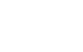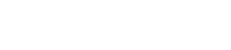## PHYSICS PAPER 3 - FORM 4 END TERM 1 EXAMS 2020

INSTRUCTIONS TO CANDIDATES

2. Answer all the questions in the spaces provided.
3. You are supposed to spend the first 15 minutes of the 2 ½ hours allowed for this paper reading the whole paper clearly before commencing your work.
4. Non – programmable silent electronic calculators and KNEC mathematical tables may be used.
5. Candidates are advised to record their observations as soon as they are made.

QUESTION 1

This question consists of part A and B. Attempt both parts.

PART A

You are provided with the following:

• A bare copper wire of diameter 0.71 mm (SWG 22) and length 50cm.
• A retort stand, boss and clamp
• An optical pin mounted on a cork
• A stop watch
• Wire cutters /pliers(to be shared)
• A metre rule or half metre rule

Proceed as follows:

1. Clamp the cork so that optical pin is horizontal. Hang the copper wire from the pin by the loop as shown in figure 1. Ensure the wire is straight and the length X between the lower tip and the optical pin is 32 cm. if the length exceeds 32 cm reduce by cutting at the lower tip using the wire cutters provided.2. Displace the lower tip of the wire slightly in a plane perpendicular to the optical pin and then release it. Measure the time t=10 oscillations of the wire and record the value in table 1.
3. Repeat the procedure in (b) above for other values of X shown in the table. (Note that each length X is obtained by cutting off an appropriate length from the lower tip of the wire. For example, to get X= 28 cm cut off 4 cm from the lower end). Complete the table. (5 Marks)
 Length X cm 32 28 24 20 16 12 Time t for 10 oscillations (s) Period T= (s) T2 (S2)
4. Plot a graph of T2 (y-axis) against X (metres) on the graph paper provided. (5 marks)
5.
1. Determine the slope, S, of the graph.             (3 marks)
2. Obtain the value of K in the equation S=  /3k(2marks)

PART B

You are provided with the following:

- A cylindrical container (about 20cm high and diameter 8cm or more)- used plastic containers can be used by cutting the upper section

- Some water

- A stop watch

- A metre rule or half-metre rule

- A boiling tube

- Some sand (in 100ml beaker)

- Spatula

- A rubber band

Proceed as follows:

1. Tie the rubber band round the boiling tube so that it is at a distance L =12 cm from the bottom of the tube (see fig 2a). Pour water into the cylindrical container until the level is about 2.0 cm from the top of the beaker. Float the boiling tube in the water in the container. Add sand gradually into the boiling tube until the tube sinks to the 12 cm mark. See figure 2(b).Depress the boiling tube slightly and release so that it oscillates vertically without touching the sides of the container. Measure and record in table 2 the time t1, for five oscillations of the boiling tube. Repeat the procedure two more times to obtain t2 and t3 and record the values in table 2. Complete the table.         (3 marks)2. Evaluate P= 40L /T2given that L is the length of the tube in metres up to the rubber band in part (f) and T is the value obtained in (g) above. (2 marks)
P=

QUESTION TWO

You are provided with the following.

- A 250 cm3 beaker

- Water

- a metre rule

- Screen

- Candle

1. Add 200cm3 of water to the vessel and obtain ‘h’ the height in centimetres of the water above the base of the vessel. Determine the appropriate value of R, the internal radius in centimetres from the formulae;
h= _________________________ cm                                                                                   (1 Marks)R=_______________________cm                                                                                        (1 Marks)
This experiment uses a cylindrical vessel, filled with water as a lens and compare its radius with the effective focal length.
2. Set the apparatus as shown in diagram below:Set u to be about 10R away from the Centre of the ‘lens’ and adjust the position of the screen to locate the image formed. The image is a sharp vertical line. Measure u and v from the Centre of the vessel. Repeat the experiment with the follow multiples of R. and record all values of u and V in the table below:                                                                                                                             (8 marks)
 10R 9R 8R 7R 6R 5R 4R 3R U (cm) V (cm)
NB: Any other appropriate value of u depending on the value of R obtained can be awarded.
3. Plot a graph of u (cm) against v (cm).             (5 marks)
4. From the graph determine
1. ‘V’ the value of V for which v=u             (1 Mark)
2. ‘U’ the value of U for which u=2v (1 Mark)
5. Determine the effective focal length of the ‘lens’ from the formulae f=('U' + 'V')/5 (2 Marks)
6. Hence determine the value of  R/f(1 Mark)## Marking Scheme

1. Table 1
 Length X cm 32 28 24 20 16 12 Time t for 10 oscillations (s) 9.32 8.72 8 7.4 6.62 5.75 Period T= (s) 0.932 0.872 0.8 0.74 0.662 0.575 T2 (S2) 0.87 0.76 0.64 0.55 0.44 0.33
(any 4 to 6 correct values, 2 marks, 2 to 4 values, 1 mark, less than 2 values, no mark)
2. Plot a graph of T2 (y-axis) against X (metres) on the graph paper provided. (5 marks)Scale =1 mark
Axes with units=1 mark
Plots = 2 marks
Line = 1 mark
3.
1.(3 marks)
2. Obtain the value of K in the equation S= (2marks)PART B

1.(3 marks)
2.(2 marks)

QUESTION TWO

1.(2 Marks)
2.                                                                                          (8 marks)
 10R 9R 8R 7R 6R 5R 4R 3R U (cm) 39.8 35.9 31.9 27.9 23.9 19.9 15.9 12.0 V (cm) 8.5 8.8 9.1 9.1 10.4 10.6 11.4 15.0
NB: Any other appropriate value of u and v depending on the value of R obtained can be awarded.
-each correct value = ½ mark
3.
(5 marks)
Scale =1 mark
Axes with units=1 mark
Plots = 2 marks
Smooth Curve = 1 mark
4. From the graph determine
1. ‘V’ the value of V for which v=u                                                                                          (1 Mark)
‘V’=13cmP
2. ‘U’ the value of U for which u=2v (1 Mark)
‘U’ = 10.4cmP
5. Determine the effective focal length of the ‘lens’ from the formulae f=('U' + 'V')/5(2 Marks)6. Hence determine the value of (1 Mark)#### Download PHYSICS PAPER 3 - FORM 4 END TERM 1 EXAMS 2020.

• ✔ To read offline at any time.
• ✔ To Print at your convenience
• ✔ Share Easily with Friends / Students